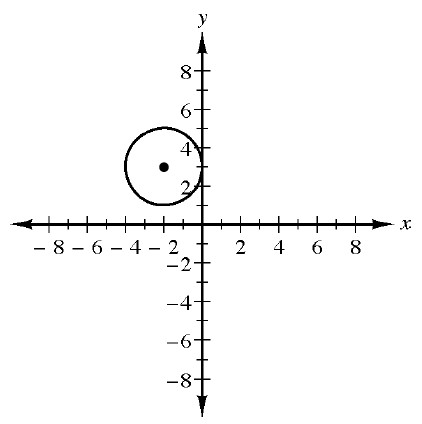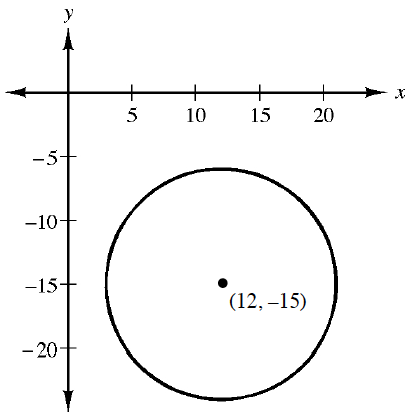### Home > CCA2 > Chapter 2 > Lesson 2.2.5 > Problem2-165

2-165.

Write an equation for each of the circles shown in the graphs below.

1.$(x-h)^2+(y-k)^2=r^2$

Where is the center? How long is the radius?

$(x+2)^2+(y-3)^2=4$

1.Refer to part (a).

Explore using the eTool below.
Click the link at right for the full version of the eTool: CCA2 2-165 HW eTool